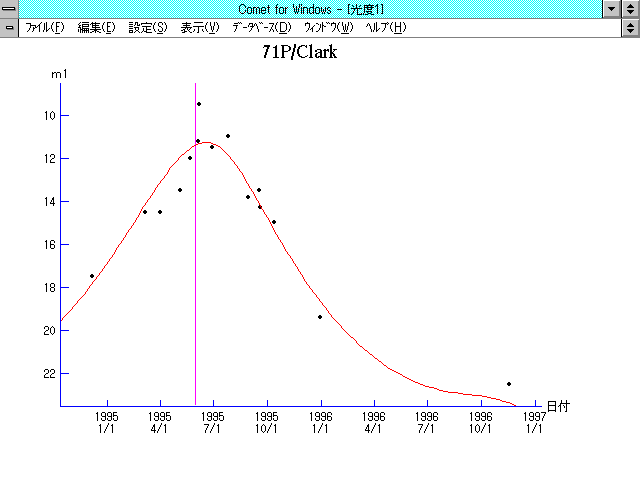\$B%/%i!<%/WB@1(B

71P/Clark (1995)\$B50F;MWAG(B

The following improved orbital elements by Kenji Muraoka,
are from 182 observations 1989 to 1997, including 9 Planets,
Moon and 5 minor planets perturbations and non-gravitational
effect of style II.  The mean residual is +/- 0.81 arc seconds.

Epoch  =  1995 June 12.0  TT       JDT = 2449880.5
T  =  1995 May  31.09704       +/- 0.00650 (m.e.) TT
Peri. =  208.84913                +/- 0.00155
Node  =   59.72323                +/- 0.00009   (2000.0)
Incl. =    9.50496                +/- 0.00002
q  =    1.5525802              +/- 0.0000028 AU
e  =    0.5019746              +/- 0.0000024
a  =    3.1174719              +/- 0.0000052 AU
n  =    0.17906069             +/- 0.00000045
P  =    5.504                  +/- 0.0000137  years
A1  =   +0.995                  +/- 0.116
A2  =   -0.54333                +/- 0.01013\$B@1?^(B1994\$BG/(B11\$B7n(B 4\$BF|!A(B1995\$BG/(B 9\$B7n(B20\$BF|(B1995\$BG/(B 9\$B7n(B20\$BF|!A(B1996\$BG/(B 3\$B7n(B18\$BF|(B\$B8wEYJQ2=(B

m1 = 8.0 + 5 log\$B&\$(B + 22.0 log r\$B50F;MWAG\$OB<2,7r<#;a\$N7W;;\$K\$h\$k\$b\$N\$G\$9!#(B \$B@1?^\$O(B StellaNavigator Ver.2.0 for Windows (\$B%"%9%H%m%"!<%D(B \$BJTCx(B / \$B%"%9%-!<=PHG6I4)(B) \$B\$G:n@.\$7\$?\$b\$N\$G\$9!#(B \$B8wEY%0%i%U\$O(BComet for Windows\$B\$G:n@.\$7\$?\$b\$N\$G\$9!#(B